#Quality Digest

Featured Product
This Week in Quality Digest Live
Quality Insider Features
Annette Franz
You may think you are, but most are probably not
Kevin Price
Turning to smart technologies to keep mission-critical equipment running—no matter what
Venkat Viswanathan
It’s not just about energy
Amadou Diallo
Cash-strapped schools are selling bonds to buy students laptops
Shobhendu Prabhakar
Even in well documented systems, people are the weakest link
Quality Insider News
Gain visibility into real-time quality data to improve manufacturing process efficiency, quality, and profits
Identify leaks in cooling tubes, hoses, and plates; prismatic battery cells and modules; battery pack assemblies
Met with students to teach them about metrology using the Artec Leo and Geomagic Design X
Deep Reality Viewer creates stereo, high-definition 3D images without using a monitor
Total focusing methods exceed conventional imaging by optimally focusing at each point of the reconstruction zone
Simple, portable, easy-to-perform calibration management with zero training
iGPS technology enables advanced manufacturing and assembly of large-scale products
Wing’s shape shifts automatically for aerodynamic loads (e.g., takeoff, landing, cruising)
Makes it faster and easier to find and return tools to their proper placesBio

Quality Insider

## Beware the Tukey Control Chart

### Another bad idea surfaces

Published: Monday, August 5, 2013 - 16:06

I recently read about a technique for analyzing data called the "Tukey control chart." Since Professor John Tukey is no longer with us, it appears that someone without his brilliance has tried to adapt one of his techniques into an alternative type of control chart. To understand the inappropriateness of this approach, read on.

John Tukey was one of the foremost statisticians of the 20th century. He created many profound techniques for analyzing data of every type. So let me be clear that none of what follows is in any way a critique of Dr. Tukey or his work. However, when others adapt a technique for use in a way in that it was never intended to be used, and when that adaptation does not work as well as the standard technique, that adaptation must be judged to be a failure.

This is the case with the Tukey control chart.

### The traditional chart

The easiest way to make a comparison is to use an example. In this case I will use 25 samples of 50 colored beads drawn from a bead box containing approximately 5,000 beads. These data come from a classroom experiment where different students took turns drawing samples of 50 beads at a time using a paddle having 50 holes on one side. We counted the number of yellow beads in each sample of 50 beads, and recorded the observed counts. The data for these 25 draws are: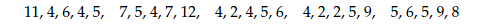The traditional X chart uses the average as the central line. The average of these 25 counts is 5.64. To complete the computations for our X chart, we find the differences between the successive values. These 24 differences are: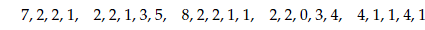The average of these 24 "moving ranges" is 2.54. To compute limits for our X chart we multiply this average moving range by the scaling factor of 2.66, and then add and subtract this product to and from the average count of 5.64.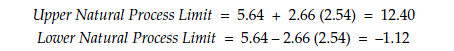Since counts cannot be negative, this lower limit is replaced by the boundary value of zero. Notice the simplicity of the computations. The differences could be found without the aid of a calculator. We compute two averages, multiply by a constant, and perform an addition and a subtraction. This simplicity is intentional because these techniques were developed and used without the aid of computers and calculators. The X chart for these counts is shown in figure 1.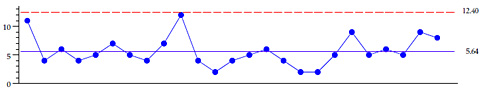Figure 1: X chart for counts of yellow beads in 25 samples of 50 beads

In this case, all 25 of the counts fall within these limits, and we fail to find any evidence of a change in the process. This was expected since the beads were placed back in the bead box after each draw, leaving the percentage of yellow beads unchanged during the course of this experiment. Drawing beads with replacement is a prototype of a predictable process. If these counts were not predictable, then no process could ever be expected to be predictable. My classes today get the same results that my classes got 30 years ago.

### The Tukey control chart

John Tukey had nothing to do with the following technique. As we will see, the Tukey control chart is only loosely based on an analytic technique developed by Tukey. It uses the median value as the central line. To find the median of a set of numbers, you will have to place these numbers in numerical order. To place more than a handful of numbers in numerical order, you will need to create a tally plot. The tally plot for the 25 counts of yellow beads given earlier is shown in figure 2.Figure 2: Tally of the counts of yellow beads

Next, this tally is used to place the counts in numerical order: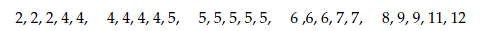Once we have the data arranged in order, we find the middle value of these 25 values to be the 13th value from either end. This median value is 5.

To characterize the variation within the data, the Tukey control chart uses the "interquartile range" (IQR). This is the difference between the upper quartile and the lower quartile for the data. Thus, we now have to find the quartiles for the data. You do remember how to do this, don't you? Well, don't worry; it is fairly easy once you have arranged the data in numerical order.

For an odd number of data points, the lower quartile is the median of the lower half of the data, including the median. For an even number of data points, the lower quartile is the median of the lower half of the data. For our data set with 25 values, the lower quartile will be the middle value of the lower 13 values. This lower quartile is the 7th value from the bottom, which is 4.

For an odd number of data points, the upper quartile is the median of the upper half of the data, including the median. For an even number of data points, the upper quartile is the median of the upper half of the data. For our data set with 25 values, the upper quartile will be the middle value of the upper 13 values. This upper quartile is the 7th value from the top, which is 7.

Thus, the interquartile range is IQR = 7 – 4 = 3. This inner quartile range is then multiplied by 1.5, and the product is added to the median and subtracted from the median to get the limits for the Tukey control chart.Figure 3: Tukey control chart for counts of yellow beads in samples of 50 beads

In figure 3 we find that two of the 25 counts fall above the upper limit. So what do these two points tell us? Has a change occurred? Did the percentage of yellow beads magically change during the course of this experiment? No, of course not. These two points have to be interpreted as false alarms, and figure 3 illustrates one of the inherent weaknesses of this approach. The limits used in the Tukey control chart are much tighter than those used by the traditional X chart. In fact, they are essentially two-sigma limits. To learn how two-sigma limits will always increase the false alarm rate, see my article "Contra Two Sigma" in the May 1, 2013, edition of Quality Digest Daily.

The data used in figures 1 and 3 are just the first 25 counts out of a set of 200 such counts all drawn from the same bead box in the same manner, with replacement, using the same paddle. Such data are, by definition, predictable and unchanging. Figure 4 shows the full set of 200 counts as eight X charts, where the limits shown are computed from each set of 25 counts. Figure 5 shows the same 200 counts on Tukey control charts.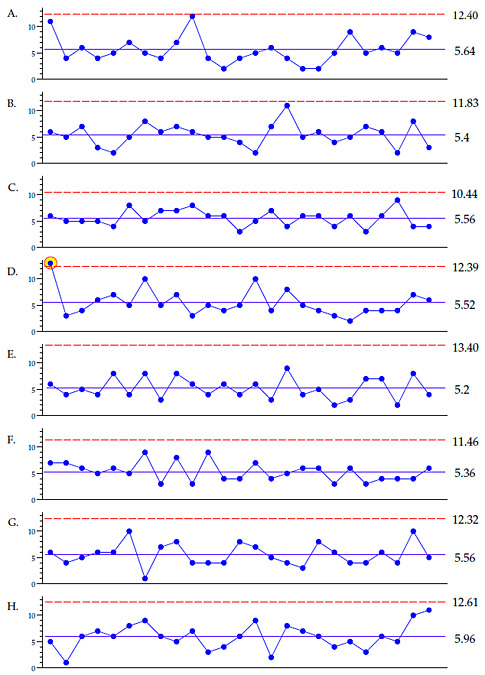Figure 4: Traditional X charts for eight sets of 25 counts of yellow beads

While the traditional X charts do have one false alarm out of 200 points, the Tukey control charts have 22 false alarms out of 200 points. While we might have expected the approximate two-sigma limits of the Tukey control charts to yield about 5-percent false alarms, the uncertainty in the computations increased the false alarm rate up to 11 percent in this instance.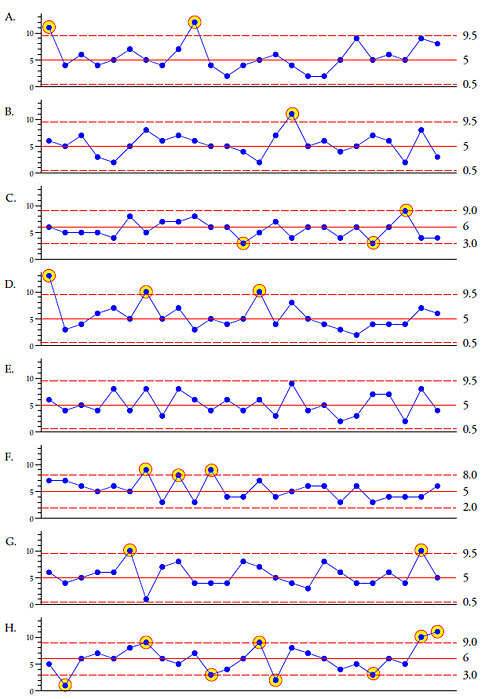Figure 5: Tukey control charts for eight sets of 25 counts of yellow beads

Thus, the computations for the Tukey control chart involve (1) the ranking of the data, (2) the finding of a median, and (3) the finding of the quartiles of the data, before you can begin to compute your limits.

This use of unfamiliar statistics along with the need to rank the data immediately make this approach more trouble than the traditional XmR chart. Secondly, the use of what are effectively two-sigma limits will inevitably result in a large number of false alarms.

The third problem with this technique is its use of inefficient summaries of location and dispersion. The median is inherently more uncertain than the average. This is why it is only used as a back-up measure of location with the traditional X chart. In addition, the interquartile range is much more uncertain as a measure of dispersion than is the average moving range.

During my 43 years of teaching data analysis techniques, I have found people generally have a harder time finding a median than they do in finding an average. And when you ask them to find the quartiles, they tend to give you that "you've got to be kidding" look. So while the final steps in computing the limits are easy, the preparations for these final steps is much more complex with the Tukey control chart than with the X chart.

However, the final problem with the Tukey control chart is the fact that it uses the wrong formula.

### Tukey's fences

Many years ago John Tukey suggested a simple way to get an intuitive feel for a finite data set. He would begin by arranging the data in numerical order. Next he would find the median value and the hinge values (also known as the quartiles). He would then summarize the data using a five-number summary consisting of the minimum, the lower quartile, the median, the upper quartile, and the maximum. This quick summary is often shown graphically in what is commonly known as a box-and-whiskers plot, although Tukey preferred the "tally plot" or "stem-and-leaf plot" rather than the sterile box-and-whiskers plot. Compare these in figure 6, and you will see why.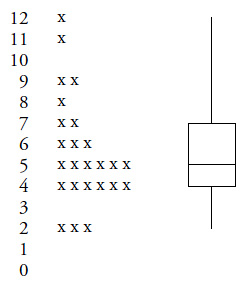Figure 6: Tally plot and box-and-whiskers plot for data set A

Then, as a refinement on this simple five-value numerical summary, Tukey used the quartiles to define "fences," and values that fell outside the inner fences were considered to be "out" while values outside the outer fences were "far out." The difference between the quartiles, known as the interquartile range or IQR, was used to compute the fences. The inner fences were 1.5 times the IQR outside the quartiles. That is, the upper inner fence is the upper quartile (UQ) plus 1.5 times the IQR, while the lower inner fence is the lower quartile (LQ) minus 1.5 times the IQR: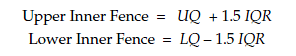Using the generic worst-case normal probability model the IQR will be approximately 1.35 standard deviations and so these inner fences will approximate the mean plus or minus 2.67 standard deviations. In terms of the analysis of a finite data set, which is the context Tukey was addressing, 2.67 sigma limits will be reasonably conservative for up to 50 or 60 data. (For data set A shown in figure 6 the observed count of 12 will be "out.") However, in terms of a sequential analysis procedure such as a control chart, experience has shown that we need wider limits in order to have an appropriately conservative procedure.

Finally, as estimates go, Tukey's fences will have a lot of uncertainty because the quartiles are less stable than the median, and both the medians and quartiles are much less reliable than the average and average moving range. The point to remember is that Tukey was not using them as estimates as much as he was using them to isolate unusual values within a finite data set.

### Summary

So while there is a superficial resemblance between John Tukey's inner fences and the limits of the Tukey control chart, the authors of the latter completely messed up the computations while they misapplied a one-time analysis technique to a sequential analysis problem. Although we might redefine the computations for the Tukey control chart to actually match Tukey's inner fences, this would not remedy the complexity of the computations or the inherent weakness of the statistics used.

Alternately, even if we changed the formula for the Tukey control chart from
[ Median ± 1.5 IQR ] to [ Median ± 2.25 IQR ] in order to approximate the three-sigma limits, the Tukey control chart would still not work as well as the XmR chart due to the inefficiency of the IQR as a measure of dispersion.

So, while John Tukey has a well-earned reputation as a brilliant statistician, the creators of the so-called Tukey control chart did not understand what Tukey was doing with his inner fences. They got the formula wrong, and their "simplified" computations are actually more complex and less efficient than those of the proven XmR chart. All of which make the Tukey control chart a technique to be avoided.

### About The Author### Donald J. Wheeler

Dr. Donald J. Wheeler is a Fellow of both the American Statistical Association and the American Society for Quality, and is the recipient of the 2010 Deming Medal. As the author of 25 books and hundreds of articles, he is one of the leading authorities on statistical process control and applied data analysis. Find out more about Dr. Wheeler’s books at www.spcpress.com.

Dr. Wheeler welcomes your questions. You can contact him at djwheeler@spcpress.com

## Comments

### Are the control limits the fences?

In the article, the control limits for the so called Tukey control chart are described as follows: "This inner quartile range is then multiplied by 1.5, and the product is added to the median and subtracted from the median to get the limits for the Tukey control chart."

In the GMU page that Dr. Wheeler references (in the comments section), the control limits are shown as follows:

LCL = Fourth – 1.5 * Fourth Spread

UCL = Three-Fourths + 1.5 * Fourth Spread

Essentially the fourth is the first quartile, the three-fourths is essentially the third quartile, and the fourth spread the IQR. The LCL/UCL calculations shown on the GMU page do not involve the median. The control limits rather are the inner fence values, and not based on the median. That makes them analogous to 2.7-sigma limits.

Perhaps the GMU page has changed since Dr. Wheeler wrote this column.

NT3327

### Typo in the text

There is a typo in the text. Near the beginning where the moving range values are listed. The last moving range value is listed as 16. It should be 1. It looks like you used the correct value in your calculations.

### Fixed the typo

Thanks Andrew. We have fixed the typo.

### This comes as no surprise

We are dealing with a binomial distribution (finite sample from a relatively infinite population, pass/fail similar to Deming's red bead experiment) which barely meets the requirements for the normal approximation (expect 4-6 occurrences per sample). The normal approximation is mediocre under these circumstances, and something that relies on medians and interquartile ranges is likely to be even worse. It is no surprise that points are above the upper control limit when the process is in control.

I do like the box and whisker plot because it is an excellent way to present data graphically, but it is a visual aid as opposed to a substitute for an analytic technique like ANOVA or a t test. I would definitely not use it as a basis for an X chart, as the author shows.

### Box and Whisker Plot

The box and whisker plot does not show the Data. It uses a five statistics computed from the data as a surrogate for the data. The plot gives major emphasis to the middle two quartiles, representing them by rectangles, and then completely deemphasizes the outer quartiles, representing them by lines. I have only found the box and whisker plot to be useful one time in the past 30 years.

### Who is advancing this?

Who is advancing the idea of a Tukey Control chart? I haven't heard of this before.

### The proponents

A correspondent sent me a link to a chapter in a book coming out of George Mason University in Virginia. The authors are listed as Farrokh Alemi, Duncan Neuhauser, and Nancy Tinsley. The URL is gunston.gmu.edu/healthscience/708/book/ChartDiet.htm

### ANOM like chart?

This technique sounds more like an ANOM type chart where you want a more exploratory type analysis for a finite data set?

Do we really need more techniques (inferior or otherwise) when we struggle to get folks to visualize their data using simple but powerful methods?

I don't think so.

Rich

### Tukey Control Charts

I have seen this chart mentioned a couple of times in health-care related applications.  Thanks for Dr. Wheeler for analyzing it in a very clear manner.

I agree with you,  Rich.  Why do we need more techniques when it is huge struggle just to get people to plot the data over time?  It seems so simple.

Thanks Dr. Wheeler,

Bill McNeese

www.spcforexcel.com

### Tukey' Control Chart

sir is tukey's chart is nt appropriate  or  i cant get ur point i am doing work on this chart may kindly  guide m what exactly the problem with tukey's control chart. only the problemm 3 sigma limits  please sir guide me

regard